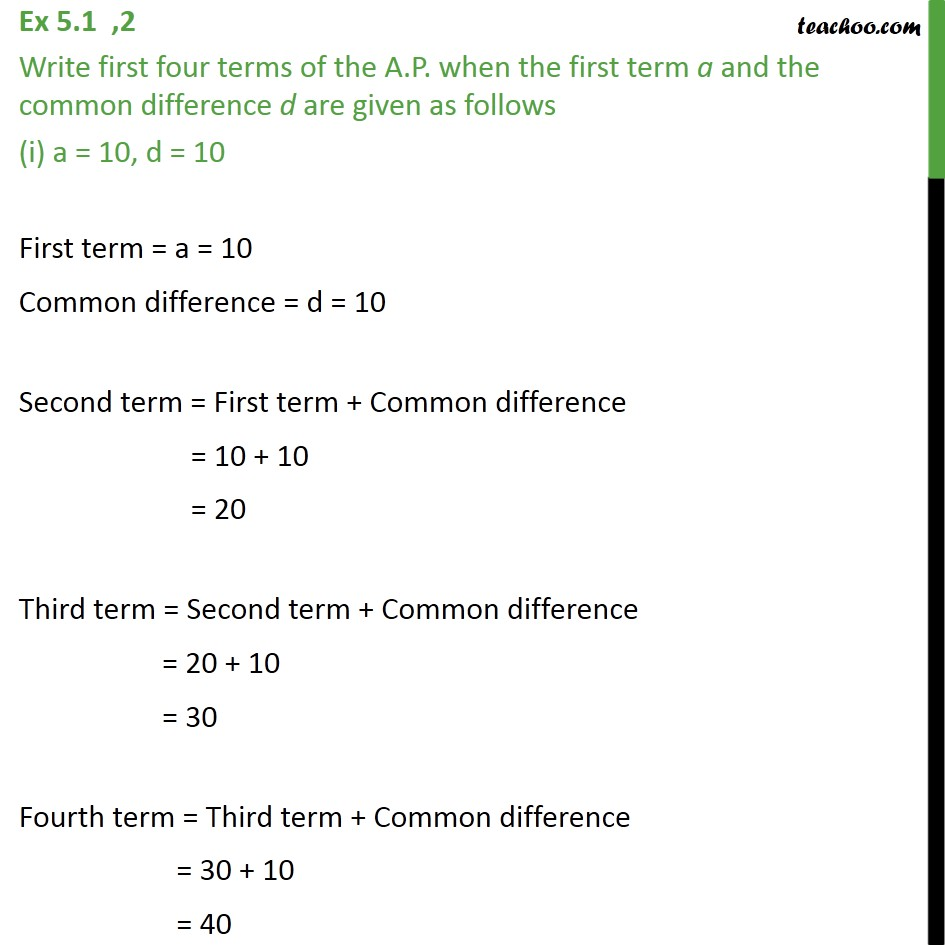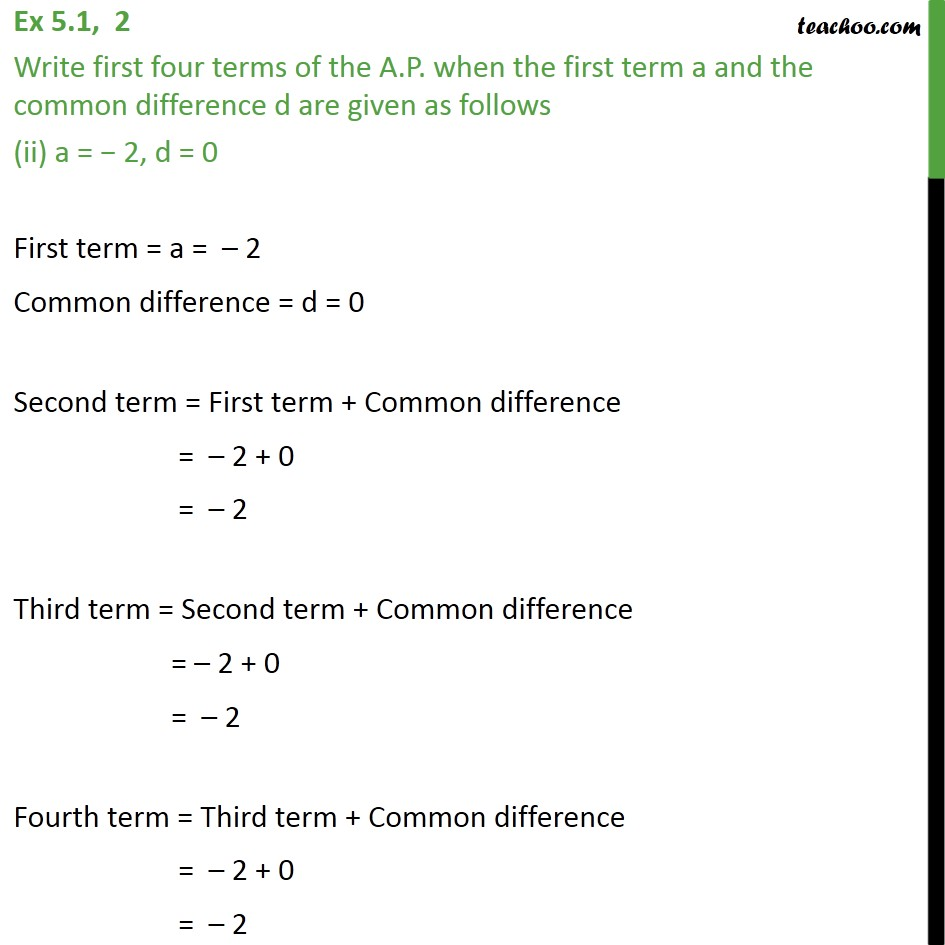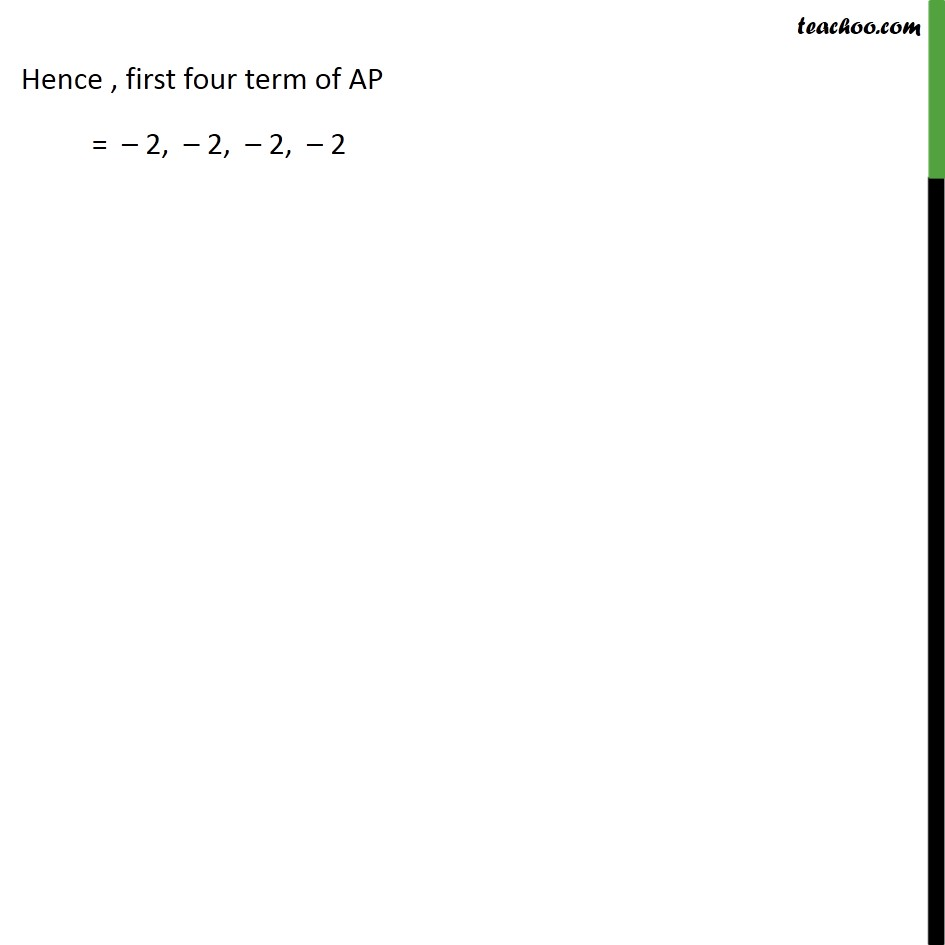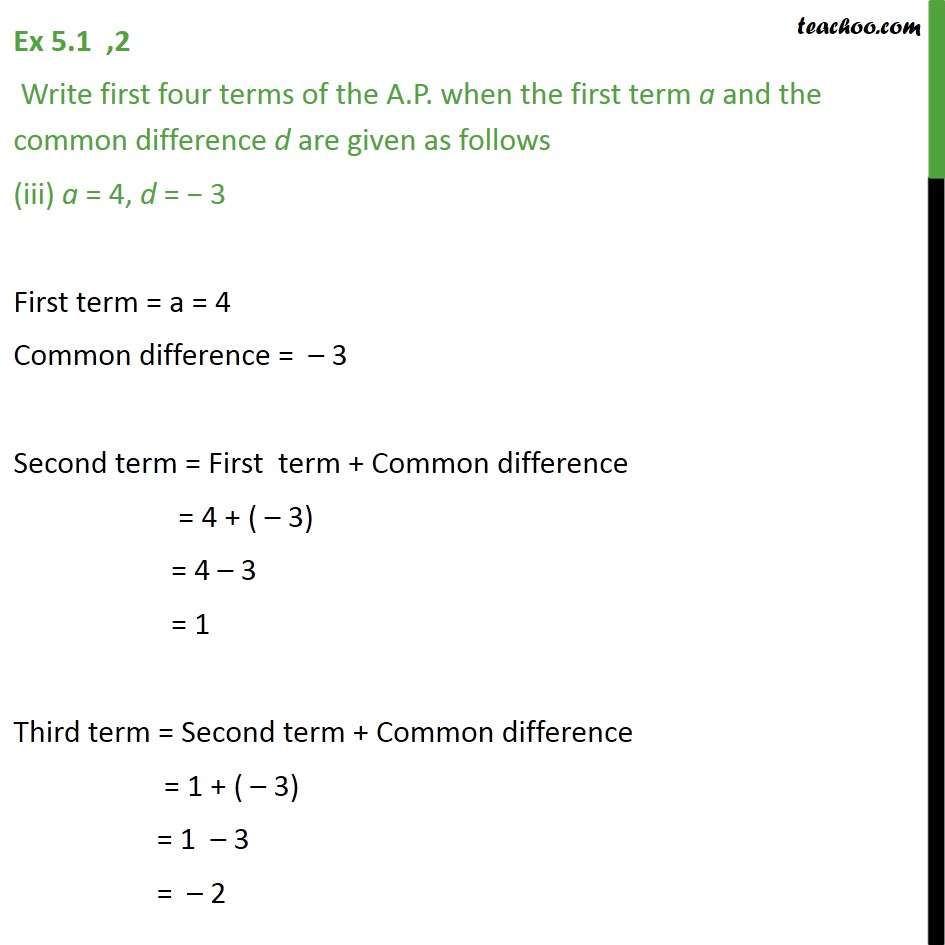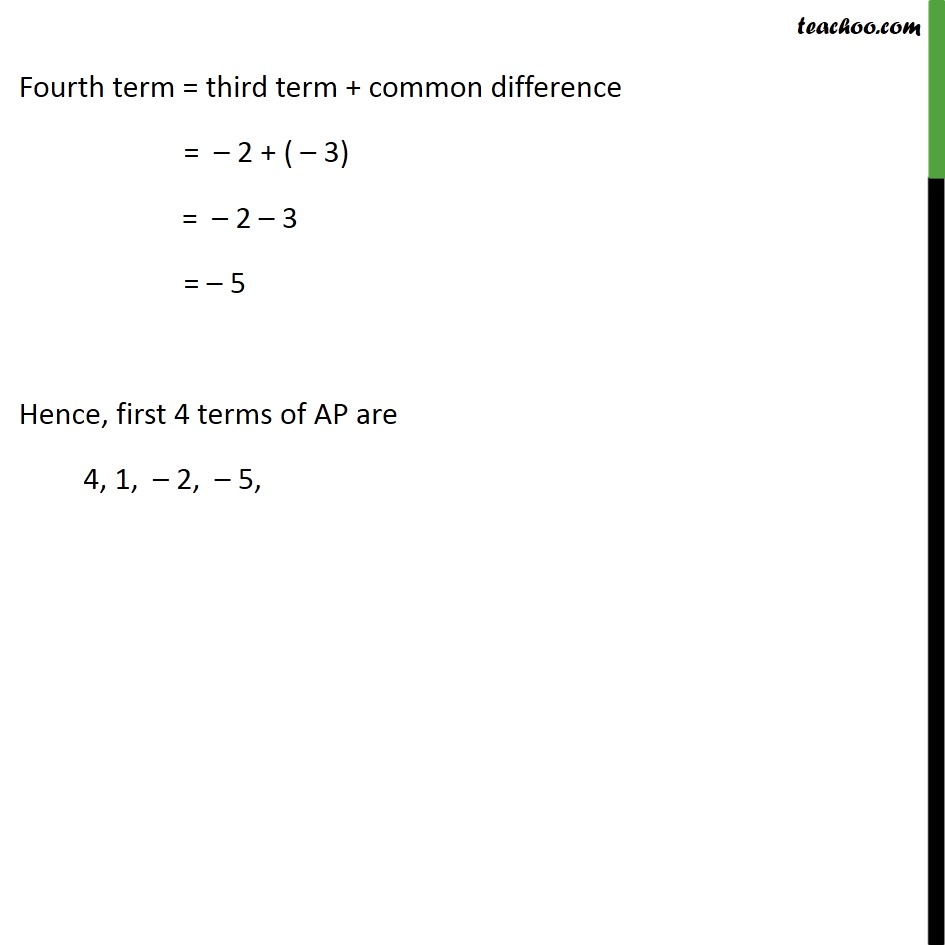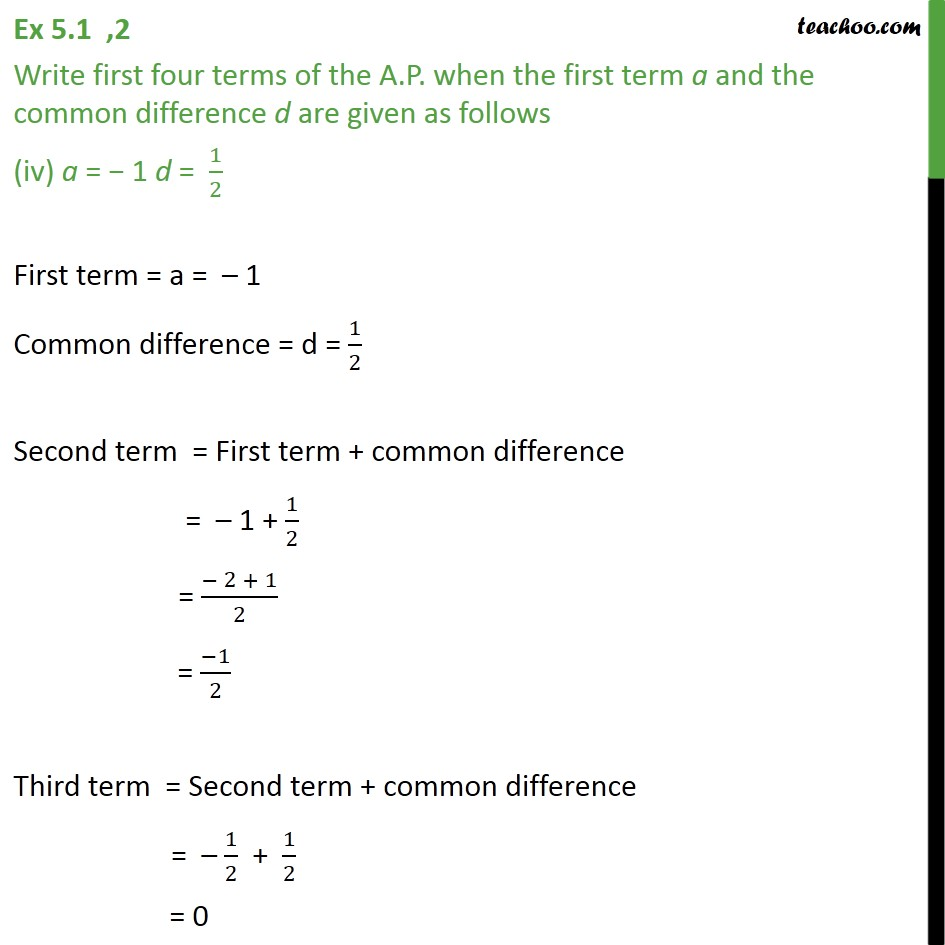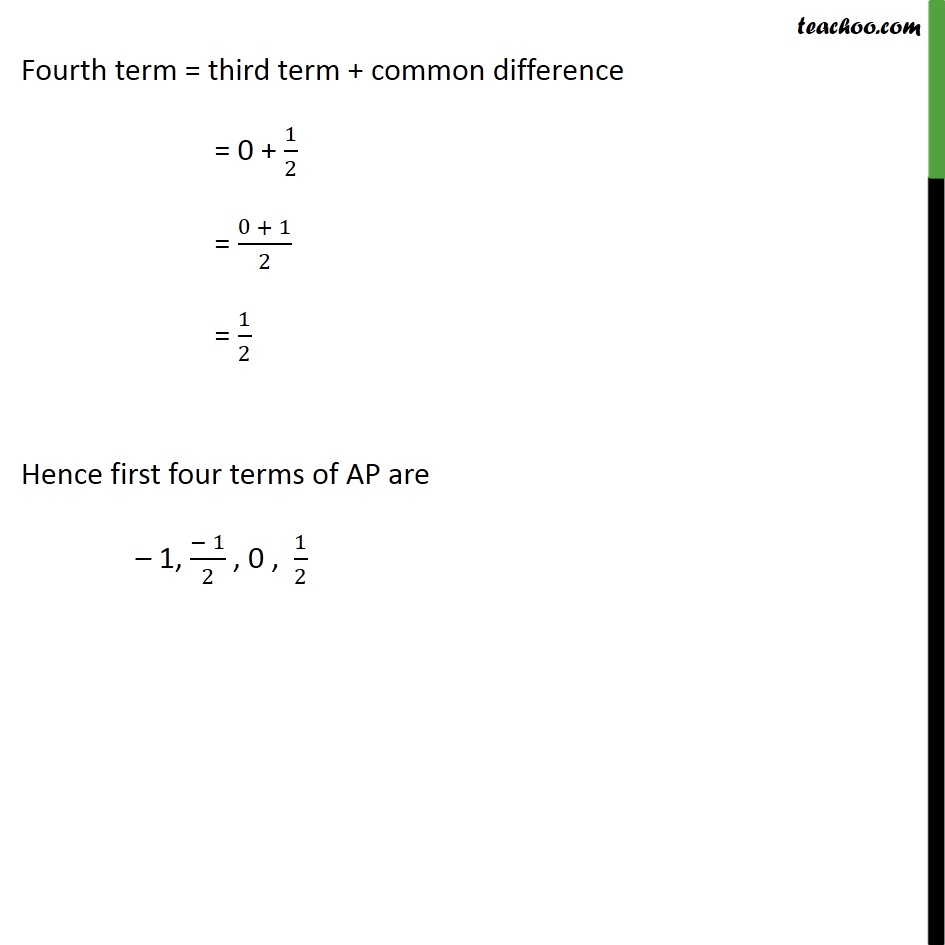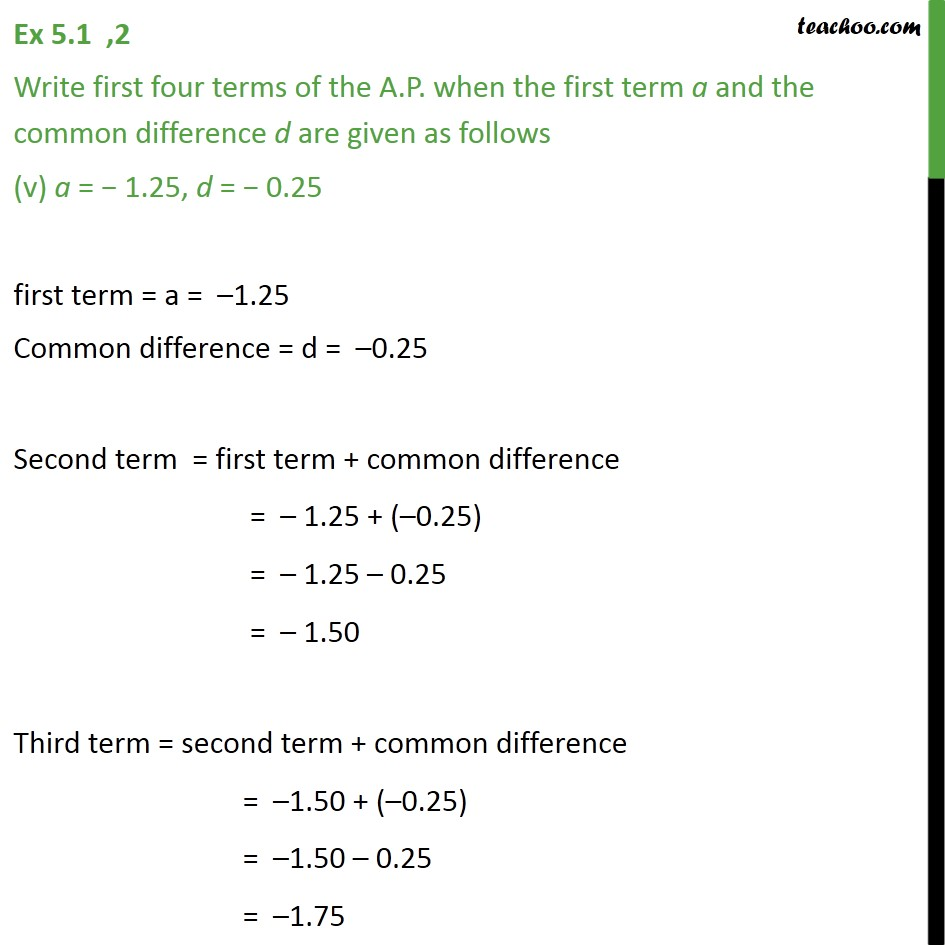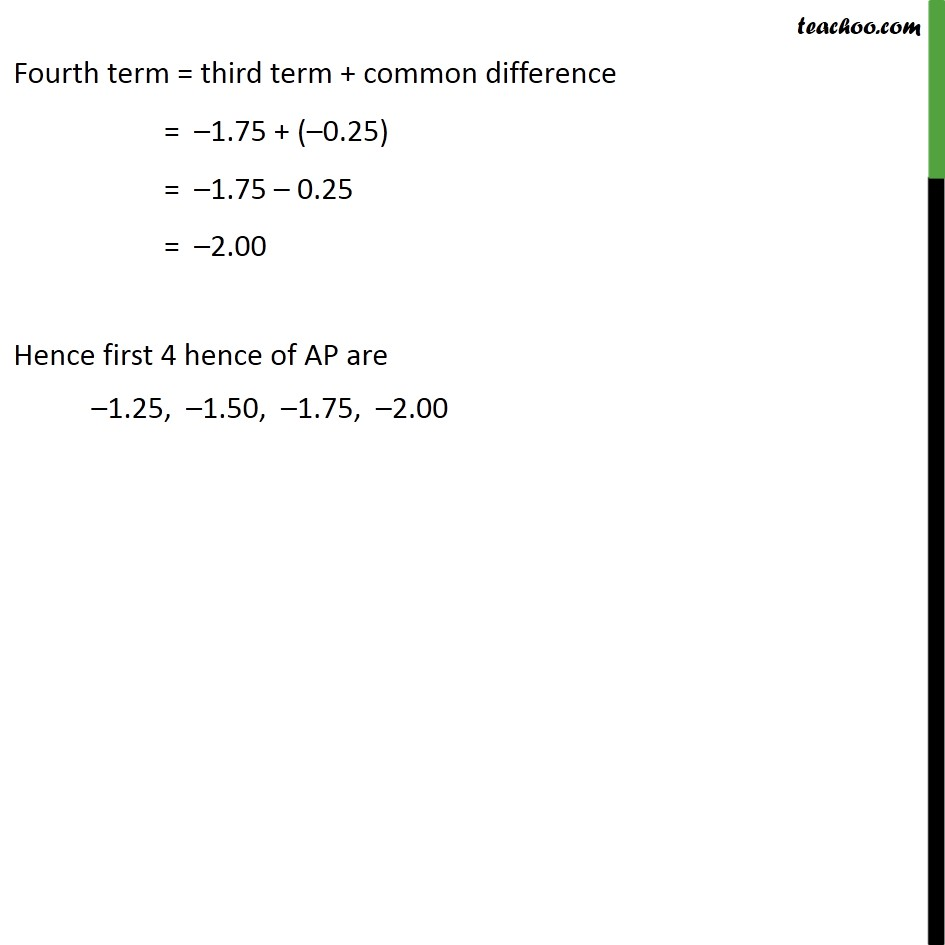1. Chapter 5 Class 10 Arithmetic Progressions
2. Concept wise
3. Checking if AP or not and finding a, d

Transcript

Ex 5.1 ,2 Write first four terms of the A.P. when the first term a and the common difference d are given as follows (i) a = 10, d = 10 First term = a = 10 Common difference = d = 10 Second term = First term + Common difference = 10 + 10 = 20 Third term = Second term + Common difference = 20 + 10 = 30 Fourth term = Third term + Common difference = 30 + 10 = 40 Hence, first four term of AP 10, 20, 30, 40 Ex 5.1, 2 Write first four terms of the A.P. when the first term a and the common difference d are given as follows (ii) a = 2, d = 0 First term = a = 2 Common difference = d = 0 Second term = First term + Common difference = 2 + 0 = 2 Third term = Second term + Common difference = 2 + 0 = 2 Fourth term = Third term + Common difference = 2 + 0 = 2 Hence , first four term of AP = 2, 2, 2, 2 Ex 5.1 ,2 Write first four terms of the A.P. when the first term a and the common difference d are given as follows (iii) a = 4, d = 3 First term = a = 4 Common difference = 3 Second term = First term + Common difference = 4 + ( 3) = 4 3 = 1 Third term = Second term + Common difference = 1 + ( 3) = 1 3 = 2 Fourth term = third term + common difference = 2 + ( 3) = 2 3 = 5 Hence, first 4 terms of AP are 4, 1, 2, 5, Ex 5.1 ,2 Write first four terms of the A.P. when the first term a and the common difference d are given as follows (iv) a = 1 d = 1/2 First term = a = 1 Common difference = d = 1/2 Second term = First term + common difference = 1 + 1/2 = ( 2 + 1)/2 = ( 1)/2 Third term = Second term + common difference = 1/2 + 1/2 = 0 Fourth term = third term + common difference = 0 + 1/2 = (0 + 1)/2 = 1/2 Hence first four terms of AP are 1, ( 1)/2 , 0 , 1/2 Ex 5.1 ,2 Write first four terms of the A.P. when the first term a and the common difference d are given as follows (v) a = − 1.25, d = − 0.25 first term = a = –1.25 Common difference = d = –0.25 Second term = first term + common difference = – 1.25 + (–0.25) = – 1.25 – 0.25 = – 1.50 Third term = second term + common difference = –1.50 + (–0.25) = –1.50 – 0.25 = –1.75 Fourth term = third term + common difference = –1.75 + (–0.25) = –1.75 – 0.25 = –2.00 Hence first 4 hence of AP are –1.25, –1.50, –1.75, –2.00

Checking if AP or not and finding a, d